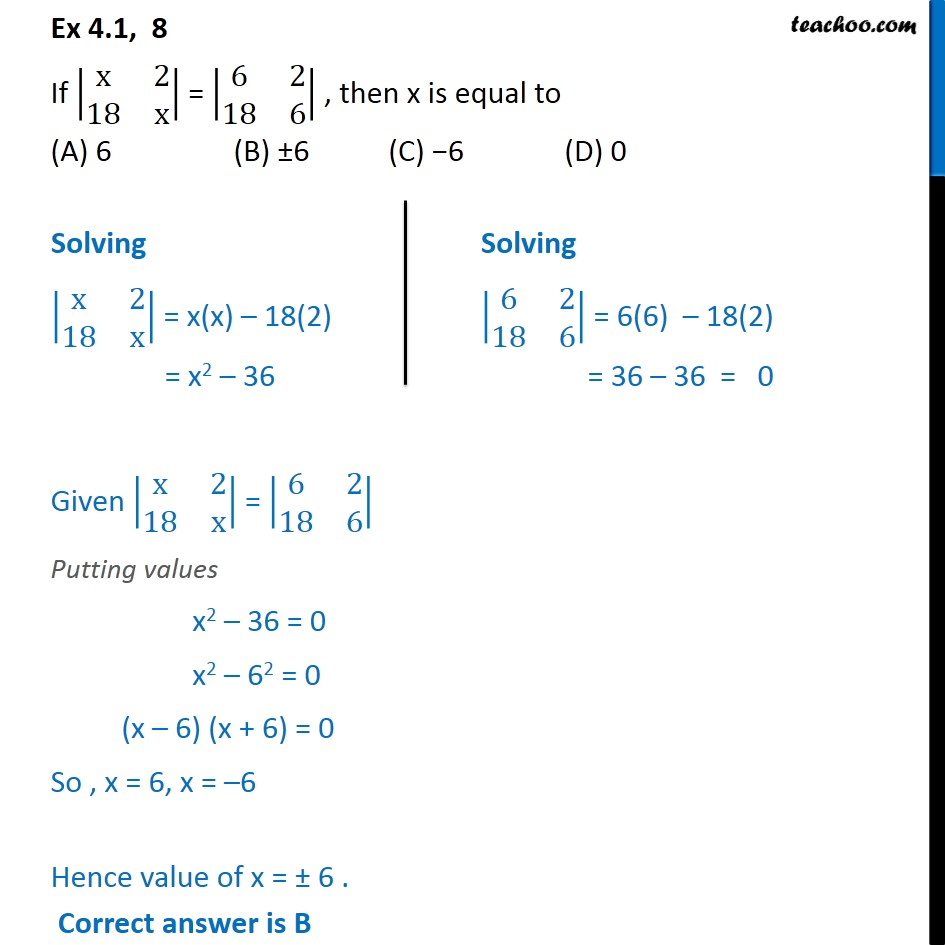1. Chapter 4 Class 12 Determinants
2. Serial order wise
3. Ex 4.1

Transcript

Ex 4.1, 8 If x 2 18 x = 6 2 18 6 , then x is equal to (A) 6 (B) 6 (C) 6 (D) 0 Given x 2 18 x = 6 2 18 6 Putting values x2 36 = 0 x2 62 = 0 (x 6) (x + 6) = 0 So , x = 6, x = 6 Hence value of x = 6 . Correct answer is B

Ex 4.1